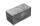# Unit conversion + cube - math problems

#### Number of problems found: 35

• Sugar cubesThe glass has 600 ml of tea, which represents 80% of the volume of the glass. If you put twenty regular sugar cubes of 2 cm in the tea, how many ml of tea are poured?
• Tower modelTower height is 300 meters, weight 8000 tons. How high is the model of the tower's weight of 1 kg? (State the result in the centimeters). The model is made from exactly the same material as the original no numbers need to be rounded. A result is a three-d
• RainfallThe annual average rainfall in India was in Cherrapunji in the year 1981 26 461 mm. How many hectoliters of water fell on 1 m2? Would fit this amount of water into a cube of three meters?
• Hectoliter into cubeCan 1hl of water fit into a half-meter cube?
• Granite cubeWhat is the weight in a kilogram granite cube with an edge of 0.5 m if 1dm3 of granite weight 2600 g?
• CubesHow many cubes with an edge length of 5 cm may fit in the cube with an inner edge of 0.4 m maximally?
• AquariumAquarium is cube with edge 45 cm. How much water can fit in there?
• Metal sheetHow much m ^ 2 of sheet metal is needed to beat a box in the shape of a cube with an edge length of 50 cm, if we add 20% on the folds of the lid and walls?
• Cube and waterHow many liters of water can fit into a cube with an edge length of 0.11 m?
• Chemical parisonThe blown parison (with shape of a sphere) have a volume 1.5 liters. What is its surface?
• Cylinder - areaThe diameter of the cylinder is one-third the length of the height of the cylinder. Calculate the surface of cylinder if its volume is 2 m3.
• Surface of the cylinderCalculate the surface area of the cylinder when its volume is 45 l and the perimeter of base is three times of the height.
• Iron sphereIron sphere has weight 100 kg and density ρ = 7600 kg/m3. Calculate the volume, surface, and diameter of the sphere.
• PrismRight-angled prism, whose base is a right triangle with leg a = 3 cm and hypotenuse c = 13 cm, has the same volume as a cube with an edge length of 3 dm. a) Find the height of the prism b) Calculate the surface of the prism c) What percentage of the cube'
• Plasticine ballPlasticine balls have radius r1=85 cm, r2=60 mm, r3=59 cm, r4=86 cm, r5=20 cm, r6=76 mm, r7=81 mm, r8=25 mm, r9=19 mm, r10=14 cm. For these balls

Do you have an exciting math question or word problem that you can't solve? Ask a question or post a math problem, and we can try to solve it.

We will send a solution to your e-mail address. Solved examples are also published here. Please enter the e-mail correctly and check whether you don't have a full mailbox.

Unit conversion - math problems. Cube Problems.